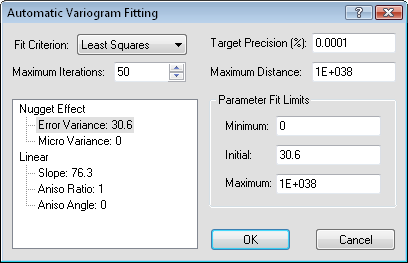# AutoFit

AutoFit takes a user-specified variogram model and an initial set of parameters, and attempts to find a better set of parameter values. For example, if you have selected a spherical variogram model with a nugget effect, the AutoFit tool can be used to determine the "best" fitting anisotropy parameters.

The AutoFit button on the Model page in the variogram properties and the Variogram page in the Grid Data dialog opens the Automatic Variogram Fitting dialog. Please note that the AutoFit tool is meant to hone and polish a set of user specified parameters. AutoFit does not select the appropriate model form. It should not be used as an exploratory tool in the search for an appropriate model. It merely fits the parameters once you select a reasonable model.

The underlying fitting algorithm is a simple implementation of Powell's Search Method. This search does not involve the use of derivatives. This means there are no gradient calculations. One iteration of this algorithm includes a line search along each of the individual parameter directions, and then a line search along the accumulated direction moved during this iteration. There is some useful discussion of this method in Press et al.(1988, Section 10.1). However, this routine does not attempt to build a set of conjugate directions.

While the fitting algorithm is working, the current iteration number and the current value of the objective function are printed on the status bar. The fit algorithm can take some time to work.The Automatic Variogram Fitting dialog is opened by clicking the AutoFit button on the Model page of the Variogram Properties dialog. The AutoFit feature does not select an appropriate model. Before using AutoFit, select reasonable model parameters and then use AutoFit to fine tune the model.

## Fit Criterion

There are two fit criteria available in AutoFit, Least Squares and Least Absolute Value. An important consideration is that both of these criteria work by fitting the model to the variogram grid in all directions at once and not to the current directional variogram plot shown on the screen.

The Least Squares criterion is an implementation of the simple weighted least squares method discussed in Cressie (1991, Section 2.6.2). See Zimmerman and Zimmerman (1991) for a rather detailed comparison among various methods. The Least Squares method minimizes the sum of the square of the error, and the Least Absolute Value method minimizes the sum of the absolute value of the errors.

The Default method uses the default linear variogram. Default calculates a slope and nugget effect similar to the default linear variogram as first displayed with New Variogram.

## Maximum Iterations

The maximum number of iterations specifies a termination criterion for AutoFit. After this number of iterations the search stops, though the iterations also terminate if a target precision is met. One iteration includes a line search for each of the free parameters, plus a line search in the direction of the accumulated change.

One feature of AutoFit is that the search can be stopped and restarted multiple times. Therefore, you could run the search for a few iterations, see what is happening, and then try a few more iterations. You can terminate the search by clicking on the ESC button on your keyboard and selecting the Abort button in the dialog that appears. If you stop AutoFit, the best set of parameters so far is saved.

## Target Precision (%)

The target precision is also a termination criterion for the search with AutoFit. The target precision specifies a maximum relative change in the objective function during one iteration. If the change in the objective function is less than the specified amount, the search is terminated. If the change in the objective function is greater than the specified amount, the search is continued for another iteration (assuming that the maximum number of iterations has not been reached). The actual formula is

## Maximum Distance

AutoFit attempts to fit the model to the data contained in the variogram grid. The extent of the variogram grid is given by the Max Lag Distance parameter specified when the variogram grid is created with the New Variogram dialog. Often, the Max Lag Distance specified when the variogram grid is created is greater than the maximum lag distance that a user is interested in working with while fitting. (Remember that the variogram grid is created before you start modeling).

The Maximum Distance parameter limits the extent of the search. The fitting algorithm ignores any pairs separated by more than the Maximum Distance, even if they are included in the variogram grid.

## Parameter Fit Limits

TheParameter Fit Limitsdefine the range of values over which the fitting algorithm searches with AutoFit. TheParameter Fit Limitsallow the user a great deal of control in applying the fitting algorithm. For example, if you specify theMaximumequal to theMinimum,the fitting algorithm does not adjust the specified parameter.

Although the fit algorithm attempts to fit parameters with a large range between the Maximum and the Minimum, you will have greater success with the fitting algorithm if the limits are as tight as you can make them. Use a "reasonable value" approach in specifying the limits.

### Minimum

The Minimum is the lower bound on the fitted parameter value. No value lower than this is considered by the fitting algorithm.

### Initial

The Initial option is the guess for the parameter value. Since the objective function is non-linear, the final fitted values can be sensitive to the starting point. The objective function (fit criterion) may have numerous local minima which are distinct from the global minima.

### Maximum

The Maximum is the upper bound on the fitted parameter value. No value greater than this is considered by the fitting algorithm.